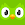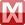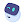# Brainly – Homework Math Solver

by Brainly
100,000,000+ installs

Android application Brainly – Homework Math Solver developed by is listed under category Education. According to Google Play Brainly – Homework Math Solver achieved more than 100,000,000 installs. Brainly – Homework Math Solver currently has 2,793,225 ratings with average rating value of 4.41. The current percentage of ratings achieved in last 30 days is 1.01%, percentage of ratings achieved in last 60 days is 2.38%. Brainly – Homework Math Solver has the current market position #357 by number of ratings. A sample of the market history data for Brainly – Homework Math Solver can be found below. Last update on 2023-01-17.

Title: Brainly – Homework Math Solver Brainly Education Free Android
Total ratings: 2793225 1.01% 2.38% 4.41
Installs (achieved): 100,000,000+ 245,676,814
5 star ratings: 2,103,953 291,207 91,827 51,951 253,937

2021-05-02: Android application Brainly – Homework Math Solver achieved 100,000,000 installs. Android application Brainly – Homework Math Solver achieved 50,000,000 installs. Android application Brainly – Homework Math Solver achieved 10,000,000 installs. Android application Brainly – Homework Math Solver achieved 5,000,000 installs. Android application Brainly – Homework Math Solver achieved 1,000,000 installs. Android application Brainly – Homework Math Solver achieved 500,000 installs. Android application Brainly – Homework Math Solver achieved 100,000 installs. Android application Brainly – Homework Math Solver achieved 10,000 installs.

Total number of ratings
Total number of active users rated for Brainly – Homework Math Solver.

Total number of installs (*estimated)
Estimation of total number of installs on Google Play. Approximated from number of ratings and install bounds achieved on Google Play.

Average rating
Average rating value on Google Play. Given by active users of the application.

Ratings count comparing to the market leading app.

Rating distribution
Rating distribution given by active users.

Related apps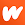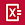Photomath
(2,858,521) [compare]